# MCAT Physical : Interference and Diffraction

## Example Questions

### Example Question #1 : Interference And Diffraction

At a local concert, a speaker is set up to produce low-pitched base sounds with a frequency range of 20Hz to 200Hz, which can be modeled as  sine waves. In a simplified model, the sound waves the speaker produces can be modeled as a cylindrical pipe with one end closed that travel through the air at a velocity of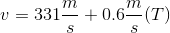, where T is the temperature in °C.

If the sound crew that set up the speakers accidently set up an additional identical speaker directly opposite the base and flipped the phase switch to produce base waves that can be modeled as cosine waves, how would the volume of the sound change at the midpoint of the two speakers?

The pitch heard increases

It becomes silent

The pitch heard decreases

It becomes quieter

It becomes silent

Explanation:

First, let’s look at what this question is asking us to consider. The question wants us to determine the relationship between waves produced from our sine phase base speaker and a cosine phase speaker accidently placed directly across from it. Two important facts come to mind when thinking about this scenario. First, the waves physically overlap in space because the speakers are pointed directly at each other. Second, the sine and cosine waves are exactly 180º out of phase. Remember that waves completely out of phase create destructive interference, meaning that the resultant wave will have no amplitude. The volume directly between the two speakers is zero because volume is directly correlated to wave amplitude.

### Example Question #2 : Interference And Diffraction

Two waves of equal amplitude destructively interfere, resulting in a wave with zero amplitude. What is the phase difference between the two waves?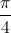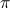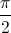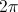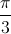Explanation:

Two waves will cancel to zero amplitude when the relative shift between them is half a period. This corresponds to half of, which gives the correct answer of.

### Example Question #3 : Interference And Diffraction

Two people are singing a note at exactly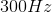. A microphone is placed in front of them to record their voices, but when the track is played back the recorded note is much quieter than the singers were producing. What phenomenon can explain this result?

The singers' combined frequency was higher than the frequency response of the microphone

The microphone was unable to pick up the sound because of the large amplitude

The waves went through destructive interference and canceled each other out

The microphone could pick up the same frequency coming from two different sound sources

The waves went through constructive interference and canceled each other out

The waves went through destructive interference and canceled each other out

Explanation:

Destructive inference occurs when the peak of one wavelength encounters the trough of another wavelength. This causes the waves to cancel each other out, essentially producing a much smaller audible wavelength. In contrast, constructive interference will result in the summation of the wave amplitudes, and an increase in the volume and intensity of the sound.

### Example Question #4 : Interference And Diffraction

A red light (wavelength = 6.5 * 10-7m) shines on a diffraction grating, creating an interference pattern on a distant screen. If a blue light (wavelength= 4.75 * 10-7m) were shone on the same screen instead, the bright lines on the screen would __________.

remain the same

become dimmer

move closer together

become brighter

move farther apart

move closer together

Explanation:

The bright lines from a diffraction grating can be located with the equation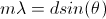, where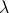is wavelength, d is the distance between slits in the diffraction grating, and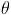is the angle of separation from the center of the interference pattern on the screen.is proportional to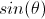, so ifdecreases, the angle of separation between lines also decreases.

### Example Question #5 : Interference And Diffraction

What term describes the phenomenon as a wave spreads into the region behind an obstruction?

Diffraction

Dispersion

Superposition

Refraction

Reflection